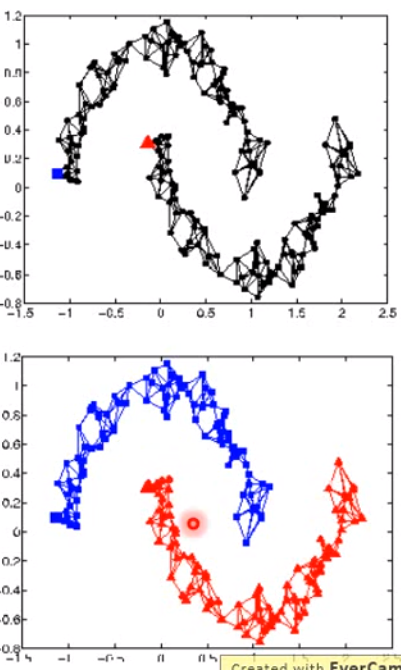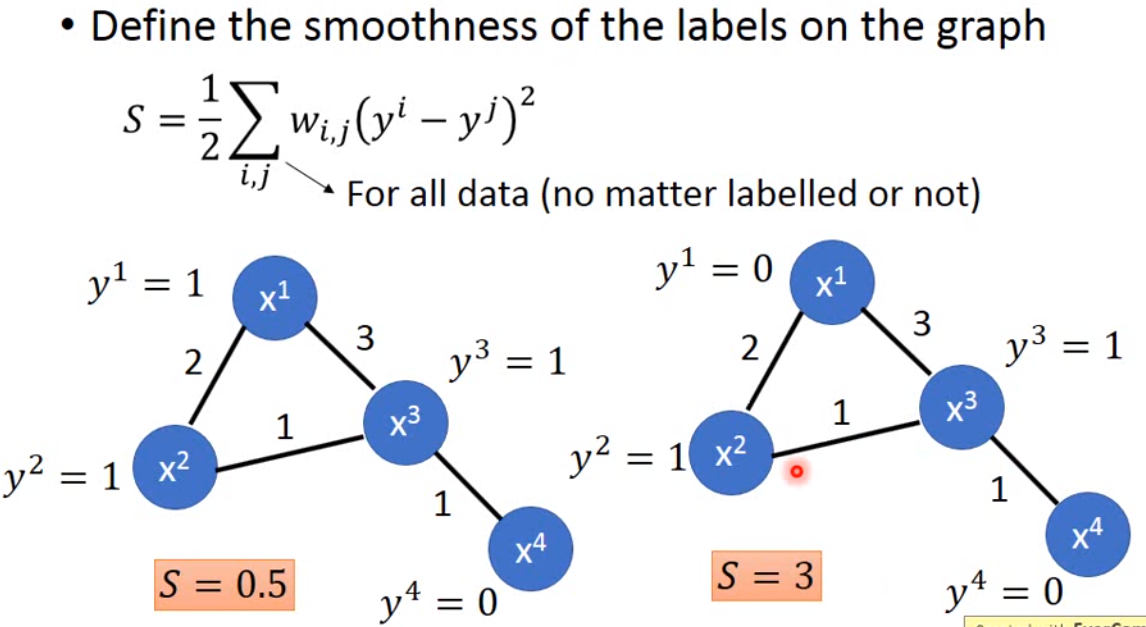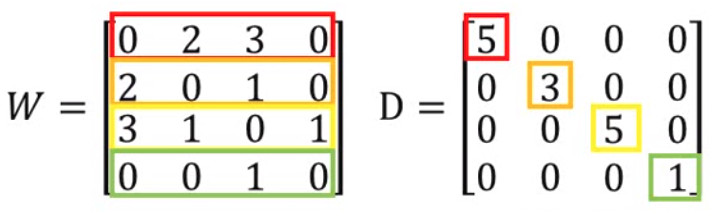semi-supervised learning并不一定总会work，这要看你的假设是否真的会成立。

# EM算法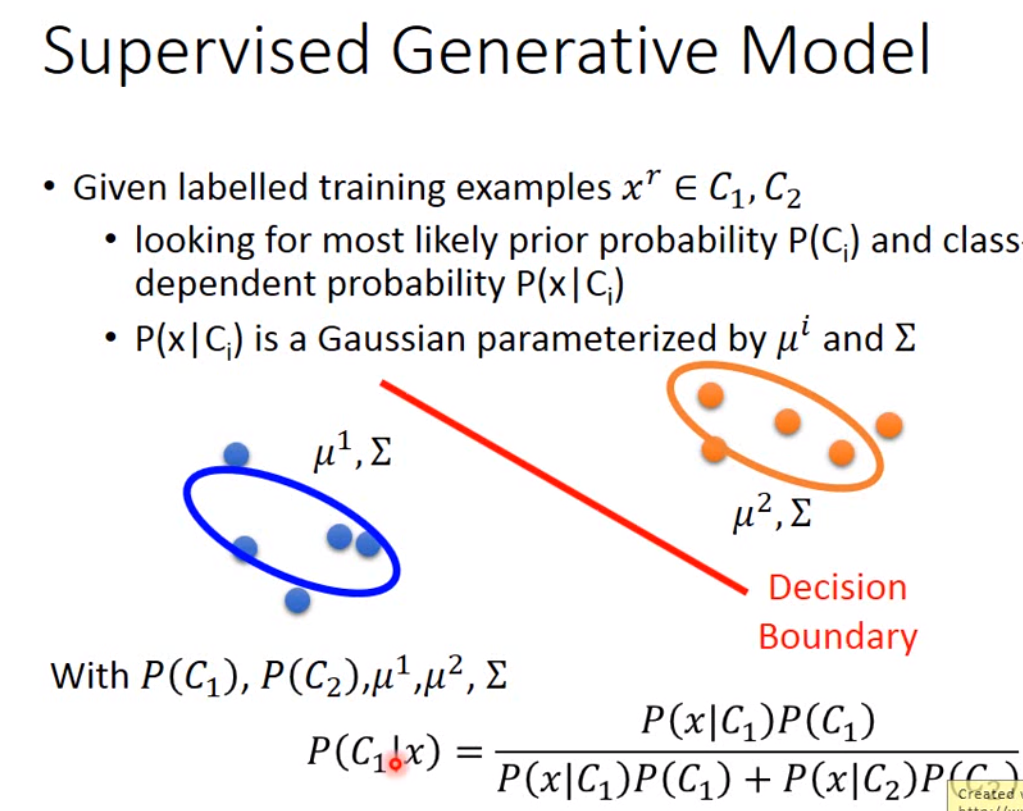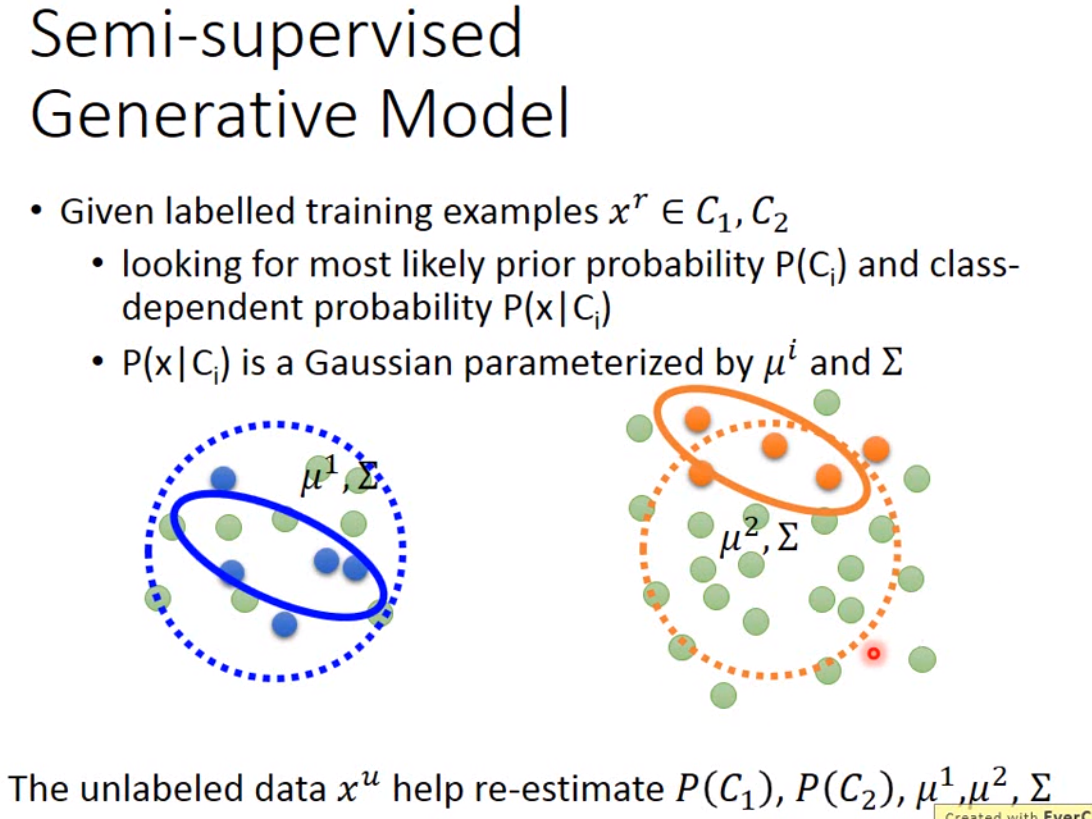• 首先初始化参数：$\theta = \{P(C_1), P(C_2), \mu^1, \mu^2, \Sigma \}$

• 其次计算unlabeled data的后验概率：$P_{\theta}(C_1 | x^u)$

• 然后更新模型：$P(C_1) = \frac{N_1 + \Sigma_{x^u}P(C_1|x^u)}{N}$，其中$N$是所有的sample，$N_1$是标记为$C_1$的样本。那如果不考虑unlabeled data，原来$C_1$的概率就是$\frac{N_1}{N}$。现在我们因为考虑了unlabeled data，因此我们在计算的时候就需要加上所有unlabeled data属于$C_1$的概率。而$\mu_1 = \frac{1}{N} \sum_{x^r \in C_1} x^r + \frac{1}{\sum_{x^u} P(C_1|x^u)} \sum_{x^u}P(C_1|x^u)x^u$。然后重复更新后验概率，直到收敛。

# Low density separation

self-training有一个进阶版本，也就是entropy-based regularization。这个值用来计算我们预测结果的分布是否集中。也就是说，我们要计算$\hat{y}^u$的entropy，也就是$E(\hat{y}^u = \sum_{m=1}^M \hat{y}_m^u ln(\hat{y}_m^u))$。那我们希望这个值越小越好。越小，说明值越集中，越大说明越分散。所以我们的loss function就可以从原来的cross entropy改进为$L = \sum_{x^r}C(y^r, \hat{y}^r) + \lambda \sum_{x^u} E(\hat{y}^u)$。这个结果就可以用梯度下降来做，另外因为后面加上了unlabeled data的这个尾巴，看上去就像是之前做regularization，所以这里就叫做了regularization。

# Smoothness assumption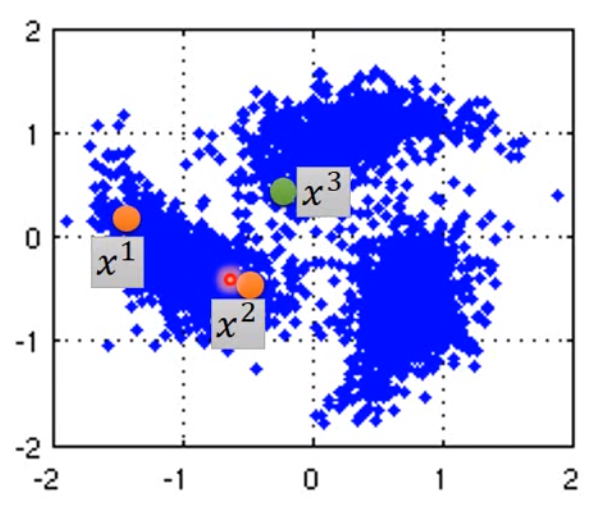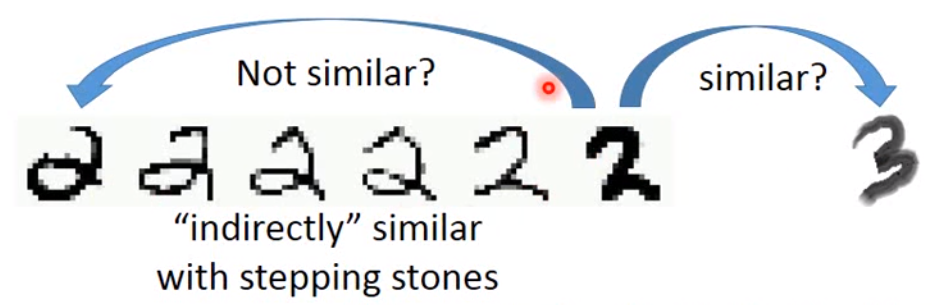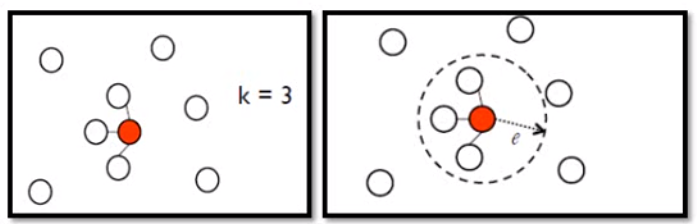KNN是连接离自己最近的K个点，而e-neighborhood是去连接半径为e内的所有点。

graph-based的方法的好处是可以将label传递出去，当然这样的做法就需要海量的数据，否则这种连接就可能断掉。如下图：